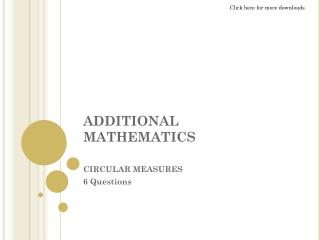# ADDITIONAL MATHEMATICS - PowerPoint PPT PresentationDownload PresentationDownload Presentation- - - - - - - - - - - - - - - - - - - - - - - - - - - E N D - - - - - - - - - - - - - - - - - - - - - - - - - - -
##### Presentation Transcript

2. Question 1 The diagram shows a sector POQ with center O and a radius 24cm. Point R and OQ is such that OR:RQ = 3.1. Calculate (a) The value of Q in radians (b) The area in cm² of the shaded region answer

3. Question 2 The diagram shows a circle with centre O and a radius of 6cm. PQR is a tangent to the circle at Q. Given PQ=QR=8cm and arc PSR is drawn with O as it centre. Calculate: (a) The angle θ in radians (b) The length in cm of arc PSR (c) The area in cm of the shaded region answer

4. Question 3 The diagram shows a sector POQ of a circle O. Point S lies on OP, point R lies on OQ and SR is perpendicular to OQ. The length of OS is 8cm and POQ=3/8 π radian. Given that OS:OP = 3:5. Calculate (a) Calculate the length of SP (b) Calculate the perimeter of shaded region (c) Calculate area of shaded region answer

5. Question 4 The diagram shows two sectors OPQR and OST with centre O and of equal area. Given that OQS and ORT are straight lines and POQ = QOR rad, calculate: (a) The radius of sector OST (b) The area of the whole shape O answer

6. Question 5 The diagram shows a sector OPQ with centre O and a radius of 10cm. Given then the area of sector is 50cm, calculate (a) The value of θ in radians (b) The length of chord PQ (c) The area of segment bounded by arc PQ and chord PQ answer

7. Question 6 The diagram shows a sector OPQR with centre O and a radius of 9cm. The length of arc PQR is 11.25cm. Calculate : (a) ∠ POR in radians (b) The area of shaded segment answer

8. Solution to Question 1 (a) Find θ in radians OR = ¾ OQ = ¾(24) = 18 cm cos θ = 18/24 = 41.41 x π/180 = 0.7227 rad (answer) (b) Area of POQ – Area of POR = ½ r²θ – ½ (OR)(PR) = ½ (24)²(0.7227) – ½ (18)(15.87) = 65.31 cm² (answer) Back to Question 1

9. Solution to Question 2 (a) tan θ = 8/6 θ = 53°13’ angle POR = 53°13’ x 2 = 106.26° 106.26° x π/180 = 1.855 rad (answer) (b) S = rθ = 10(1.855) = 18.55cm (answer) (c) ½ r²(θ – sinθ) = ½ (10)²(1.855 – sin 1.855) = 44.76 cm² (answer) Back to Question 2

10. Solution to Question 3 (a) OS = 3/5 OP OP = 5/3 OS = 5/3(9) = 15cm Given OS = 8 cm SP = OP – OS = 15 – 8 = 7 cm (answer) (c) Area of sector POQ – Area of triangle SOR = ½ r²θ – ½ (OR)(SR) = ½ (15)²(3/8 π) – ½ (3.06)(7.39) = 121.23cm² (answer) (b) S = rθ PQ = rθ = 15(3/8 π) = 17.67 cm Angle SOR = 3/8 π x 180/π = 67.5° sin 67.5° = SR/8cm SR = 7.39 cm To find RQ tan 67.5° = 7.39/OR OR = 3.06 cm RQ = OQ – OR = 15 – 3.06 = 11.94cm Perimeter = arc PQ + SP + SR + RQ = 17.67 + 7 + 7.39 + 11.94 = 44cm(answer) Back to Question 3

11. Solution to Question 4 (a) Area sector POR = Area sector SOT ½ r²θ = ½ r²θ ½ (5)²(1.6) = ½ (r²)(0.8) 20 = 0.4r² r² = 50 r = 7.071 cm (answer) (b) Area sector POR + Area sector SOT = ½ (5)²(0.8) + ½ (7.071)²(0.8) = 10 + 20 = 30 cm² (answer) Back to Question 4

12. Solution to Question 5 (a) Area of sector = 50 cm²] ½ r²θ = 50 ½ (10)²θ = 50 θ = 1 rad (answer) (b) Let the midpoint of PQ be r 0.5 x 180/π = 28.65° sin 28.65° = Pr / 10 Pr = 4.79 cm Length of chord PQ = 4.79 x 2 = 9.58 cm (answer) (c) Use ½ r²(θ – sinθ) = ½ (10)² (1 – sin 1) = 7.926 cm² (answer) Back to Question 5

13. Solution to Question 6 (a) S = rθ 11.25 = 9θ θ = 1.25 rad (answer) (b) Use ½ r²(θ – sinθ) = ½ (9)²(1.25 – sin 1.25) = 12.19cm² (answer) Back to Question 6